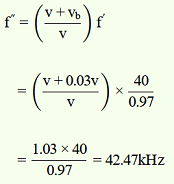# NCERT Solutions for Class 11 Physics chapter-15 Waves

## NCERT Solutions for Class 11 Physics

NCERT Solutions for class-11 Physics Chapter-15 Waves is prepared by our senior and renowned teachers of Physics Wallah primary focus while solving these questions of class-11 in NCERT textbook, also do read theory of this Chapter-15 Waves while going before solving the NCERT questions. You can download NCERT solution of all chapters from Physics Wallah in PDF.

### Chapter-15 Waves

Question1. A string of mass 2.50 kg is under a tension of 200 N. The length of the stretched string is 20.0 m. If the transverse jerk is struck at one end of the string, how long does the disturbance take to reach the other end?

Solution :
Mass of the string, M = 2.50 kg
Tension in the string, T = 200 N
Length of the string, l = 20.0 m
Mass per unit length, µ = M/l = 2.50/20 = 0.125 kg m-1
The velocity (v) of the transverse wave in the string is given by the relation:
v = √t/µ
= √200/0.125 = √1600 = 40 m/s
∴ Time taken by the disturbance to reach the other end, t = l/v = 20/40 = 0.5 s.

Question 2. A stone dropped from the top of a tower of height 300 m high splashes into the water of a pond near the base of the tower. When is the splash heard at the top given that the speed of sound in air is 340 m s–1? (g= 9.8 m s–2)

Solution :
Height of the tower, s = 300 m
Initial velocity of the stone, u = 0
Acceleration, a = g = 9.8 m/s2
Speed of sound in air = 340 m/s
The time (t1) taken by the stone to strike the water in the pond can be calculated using the second equation of motion, as:
s = ut1 + 1/2 gt12
300 = 0 + 1/2 × 9.8 × t12
∴ t1 = √300 × 2/9.8  = 7.82 s
Time taken by the sound to reach the top of the tower, t2 = 300/340 = 0.88 s
Therefore, the time after which splash is heard,  t = t1 + t2
= 7.82 + 0.88 = 8.7 s.

Question 3. A steel wire has a length of 12.0 m and a mass of 2.10 kg. What should be the tension in the wire so that speed of a transverse wave on the wire equals the speed of sound in dry air at 20 °C = 343 m s–1.

Solution :
Length of the steel wire, l = 12 m
Mass of the steel wire, m = 2.10 kg
Velocity of the transverse wave, v = 343 m/s
Mass per unit length, µ = m/l = 2.10/12 = 0.175 kg m-1
For Tension T, velocity of the transverse wave can be obtained using the relation:
v = √T/µ
∴ T = vu
= (343)2 × 0.175 = 20588.575 ≈ 2.06 × 104 N.

Question4. Use the formula v = √γP/ρ to explain why the speed of sound in air
a. is independent of pressure,
b.increases with temperature,
c. increases with humidity.

Solution :
a. Take the relation:
v = √γP/ρ          ....(i)
where,
Density, ρ = Mass/Volume = M/V
M = Molecular weight of the gas
V = Volume of the gas
Hence, equation (i) reduces to:
v = √γPV/M       ....(ii)
Now from the ideal gas equation for n = 1:
PV = RT
For constant T, PV = Constant
Since both M and γ are constants, v = Constant
Hence, at a constant temperature, the speed of sound in a gaseous medium is independent of the change in the pressure of the gas.

b. Take the relation:
v = √γP/ρ          ....(i)
For one mole of any ideal gas, the equation can be written as:
PV = RT
P = RT/V        ....(ii)
Substituting equation (ii) in equation (i), we get:
v = √γRT/Vρ  = √γRT/M  .....(iii)
where,
mass, M = ρV is a constant
γ and R are also constants
We conclude from equation (iii) that v ∝ √T
Hence, the speed of sound in a gas is directly proportional to the square root of the temperature of the gaseous medium, i.e., the speed of the sound increases with an increase in the temperature of the gaseous medium and vice versa.

c. Let vm and vd be the speed of sound in moist air and dry air respectively.

Let ρm and ρd be the densities of the moist air and dry air respectively.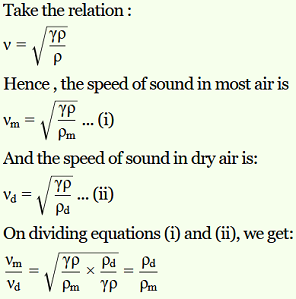However, the presence of water vapour reduces the density of air,

i.e., ρd < ρm

∴ vm > vd

Hence, the speed of sound in mois air is greater than it is in dry air. Thus, in gaseous medium, the speed of sound increases with humidity.

Question 5. You have learnt that a travelling wave in one dimension is represented by a function y = f (x, t)where x and t must appear in the combination x – v t or x + v t, i.e. y = f (x ± v t). Is the converse true? Examine if the following functions for y can possibly represent a travelling wave:
a. (x – vt)2
b. log [(x + vt) / x0]
c. 1 / (x + vt)

Solution :
No, the converse is not true. The basic requirements for a wave function to represent a travelling wave is that for all values of x and t, wave function must have finite value.
Out of the given functions for y, no one satisfies this condition. Therefore, none can represent a travelling wave.

Question 6. A bat emits ultrasonic sound of frequency 1000 kHz in air. If the sound meets a water surface, what is the wavelength of

a. the reflected sound,

b. the transmitted sound? Speed of sound in air is 340 m s–1 and in water 1486 m s–1.

Solution :
a. Frequency of the ultrasonic sound, ν = 1000 kHz = 106 Hz
Speed of sound in air, va = 340 m/s
The wavelength (λr) of the reflected sound is given by the relation:
λ= v/v
= 340/106 = 3.4 × 10-4 m.
b. Frequency of the ultrasonic sound, ν = 1000 kHz = 106 Hz
Speed of sound in water, vw = 1486 m/s
The wavelength of the transmitted sound is given as:
λ= 1486 × 10= 1.49 × 10-3 m.

Question 7. A hospital uses an ultrasonic scanner to locate tumours in a tissue. What is the wavelength of sound in the tissue in which the speed of sound is 1.7 km s–1? The operating frequency of the scanner is 4.2 MHz.

Solution :
Speed of sound in the tissue, v = 1.7 km/s = 1.7 × 103 m/s
Operating frequency of the scanner, ν = 4.2 MHz = 4.2 × 106 Hz
The wavelength of sound in the tissue is given as:
λ = v/v
= 1.7 × 103 / 4.2 × 106 = 4.1 × 10-4 m.

Question 8. A transverse harmonic wave on a string is described by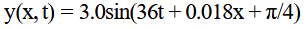Where x and y are in cm and t in s. The positive direction of x is from left to right.
a. Is this a travelling wave or a stationary wave?
If it is travelling, what are the speed and direction of its propagation?
b. What are its amplitude and frequency?
c. What is the initial phase at the origin?
d. What is the least distance between two successive crests in the wave?

Solution :
a. The equation of progressive wave travelling from right to left is given by the displacement function:
y (x, t) = a sin (ωt + kx + Φ)     ....(i)
The given equation is:......(ii)
On compaaring both the equations, we find that equation (ii) represents a travelling wave, propgating from right to left. Now using equations (i) and (ii), we can write: ω = 36 rad/s and k = 0.018 m-1 We know that: v = ω/2π and λ = 2π/k Also,
v = vλ
∴ v = (ω/2π) / (2π/k) = ω/k
= 36/0.018 = 2000 cm/s = 20 m/s
Hence, the speed of the given travelling wave is 20 m/s.

b. Amplitude of the given wave, a = 3 cm
Frequency of the given wave:
v = ω/2π = 36 / 2 × 3.14 = 573 Hz

c. On comparing equations (i) and (ii), we find that the intial phase angle, Φ = π/4

d. The distance between two successive crests or troughs is equal to the wavelength of the wave.
Wavelength is given by the relation:
k = 2π/λ

∴ λ = 2π/k = 2 × 3.14 / 0.018 = 348.89 cm =3.49 m.

Question 9. For the wave described in Exercise 15.8, plot the displacement (y) versus (t) graphs for x = 0, 2 and 4 cm. What are the shapes of these graphs? In which aspects does the oscillatory motion in travelling wave differ from one point to another: amplitude, frequency or phase?

Solution :
All the waves have different phases.
The given transverse harmonic wave is:

y(x, t) =3 sin (36t +0.018x + π/4)                . . . . . . . . .  . . ( 1 )
For x = 0, the equation reduces to:

y( 0, t ) =3 sin ( 36t +0 + π/4 )       . . . . . . . . .  . . ( 2 )
Also,
ω = 2 π/t = 36 rad/s
=> t = π/18 secs.
Now, plotting y vs. t graphs using the different values of t, as listed in the given table

 t (s) 0 T/8 2T/7 3T/8 4T/8 5T/8 6T/8 7T/8 y (cm) 3/√2 3 3/√2 0 -3/√2 –3 -3/√2 0

For x = 0, x = 2, and x = 4, the phases of the three waves will get changed. This is because amplitude and frequency are invariant for any change in x. The y-t plots of the three waves are shown in the given figure.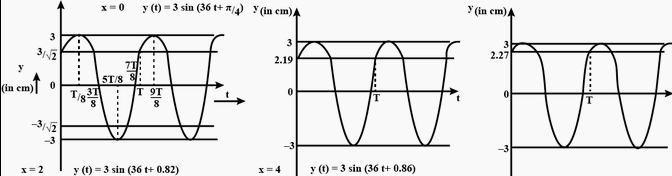Question 10. For the travelling harmonic wave y (x, t) = 2.0 cos 2π (10t – 0.0080x + 0.35) Where x and y are in cm and t in s. Calculate the phase difference between oscillatory motion of two points separated by a distance of
a. 4 m,
b. 0.5 m,
c. λ/2
d. 3λ/4

Solution :
Equation for a travelling harmonic wave is given as:
y (x, t) = 2.0 cos 2π (10t – 0.0080x + 0.35)
= 2.0 cos (20πt – 0.016πx + 0.70 π)
Where,
Propagation constant, k = 0.0160 π
Amplitude, a = 2 cm
Angular frequency, ω= 20 π rad/s
Phase difference is given by the relation:
Φ = kx = 2π/λ
a. For x = 4 m = 400 cm
Φ = 0.016 π × 400 = 6.4 π rad
b. For 0.5 m = 50 cm
Φ = 0.016 π × 50 = 0.8 π rad
c. For x = λ/2
Φ = 2π/λ × λ/2 = π rad
d. For x = 3λ/4
Φ = 2π/λ × 3λ/4 = 1.5π rad.

Question 11. The transverse displacement of a string (clamped at its both ends) is given by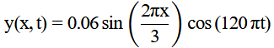Where x and y are in m and t in s. The length of the string is 1.5 m and its mass is 3.0 ×10–2 kg.

a. Does the function represent a travelling wave or a stationary wave?
b. Interpret the wave as a superposition of two waves travelling in opposite directions. What is the wavelength, frequency, and speed of each wave?
c. Determine the tension in the string.

Solution :
a. The general equation representing a stationary wave is given by the displacement function:
y (x, t) = 2a sin kx cos ωt
This equation is similar to the given equation:Hence, the given equation represents a stationary wave.
b. A wave travelling along the positive x-direction is given as:

y1 = a sin (ωt - kx)

The wave travelling along the positive x-direction is given as:

y2 = a sin (ωt + kx)

The supposition of these two waves yields:

y = y1 + y2 = a sin (ωt - kx) - a sin (ωt + kx)

= a sin (ωt) cos (kx) - a sin (kx) cos (ωt) -  a sin (ωt) cos (kx) - a sin (kx) cos (ωt)

= 2 a sin (kx) cos (ωt)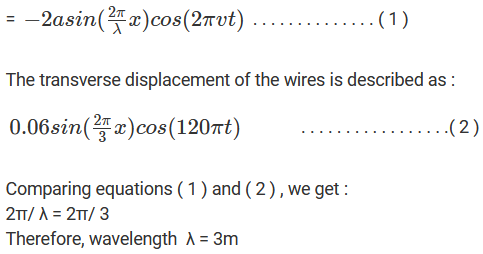∴Wavelength, λ = 3 m
It is given that:
120π = 2πν
Frequency, ν = 60 Hz
Wave speed, v = νλ
= 60 × 3 = 180 m/s
c. The velocity of a transverse wave travelling in a string is given by the relation:
v = √T/µ              ....(i)
where,
Velocity of the transverse wave, v = 180 m/s
Mass of the string, m = 3.0 × 10–2 kg
Length of the string, l = 1.5 m
Mass per unit length of the string, µ = m/l
= 3.0 × 1.5 = 10-2
= 2 × 10-2 kg m-1 Tension in the string = T
From equation (i), tension can be obtained as:
T = v2μ
= (180)2 × 2 × 10–2
= 648 N

Question 12. (i) For the wave on a string described in Exercise 15.11, do all the points on the string oscillate with the same (a) frequency, (b) phase, (c) amplitude? Explain your answers. (ii) What is the amplitude of a point 0.375 m away from one end?

Solution :
All the points on the string
(i) have the same frequency except at the nodes (where frequency is zero)
(ii) have thse same phase everywhere in one loop except at the nodes.
However, the amplitude of vibration at different points is different.

Form:

Given that,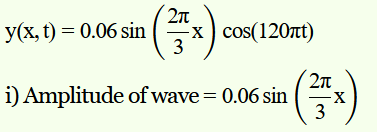Frequency =60Hz
∴ From the above equation of wave, we see that all points on the string oscillate with same phase and frequency except the nodes (endpoints).
We can also see that amplitude of the wave changes for different 'x'.
ii) Amplitude of a point at 0.375m i.e., x=0.375m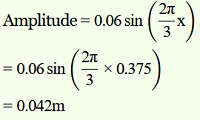Question 13. Given below are some functions of x and t to represent the displacement (transverse or longitudinal) of an elastic wave. State which of these represent (i) a traveling wave, (ii) a stationary wave or (iii) none at all:
a. y = 2 cos (3x) sin (10t)
b. y = 2 √x - vt
c. y = 3 sin (5x – 0.5t) + 4 cos (5x – 0.5t)
d. y = cos x sin t + cos 2x sin 2t

Solution :
a. The given equation represents a stationary wave because the harmonic terms kx and ωt appear separately in the equation.
b. The given equation does not contain any harmonic term. Therefore, it does not represent either a travelling wave or a stationary wave.
c. The given equation represents a travelling wave as the harmonic terms kx and ωt are in the combination of kx – ωt.
d. The given equation represents a stationary wave because the harmonic terms kx and ωt appear separately in the equation. This equation actually represents the superposition of two stationary waves.

Question 14. A wire stretched between two rigid supports vibrates in its fundamental mode with a frequency of 45 Hz. The mass of the wire is 3.5 × 10–2 kg and its linear mass density is 4.0 × 10–2 kg m–1. What is

a. the speed of a transverse wave on the string, and

b. the tension in the string?

Solution :
a.  Mass of the wire, m = 3.5 × 10–2 kg
Linear mass density, μ = m/l = 4.0 × 10-2 kg m-1
Frequency of vibration, v = 45 Hz

∴ length of the wire, l = m/μ

= 3.5 × 10–2 / 4.0 × 10-2

= 0.875 m
The wavelength of the stationary wave (λ) is related to the length of the wire by the relation:
λ = 2l/m
where,
n = Number of nodes in the wire
For fundamental node, n = 1:
λ = 2l
λ = 2 × 0.875 = 1.75 m
The speed of the transverse wave in the string is given as:
v = νλ= 45 × 1.75 = 78.75 m/s
b. The tension produced in the string is given by the relation:
T = v2µ
= (78.75)2 × 4.0 × 10–2 = 248.06 N

Question 15. A metre-long tube open at one end, with a movable piston at the other end, shows resonance with a fixed frequency source (a tuning fork of frequency 340 Hz) when the tube length is 25.5 cm or 79.3 cm. Estimate the speed of sound in air at the temperature of the experiment. The edge effects may be neglected.

Solution :
Frequency of the turning fork, ν = 340 Hz
Since the given pipe is attached with a piston at one end, it will behave as a pipe with one end closed and the other end open, as shown in the given figure.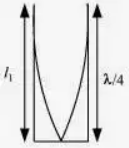Such a system produces odd harmonics. The fundamental note in a closed pipe is given by the relation:
l1 = π/4 where, length of pipe, l1 = 25.5 cm = 0.255 m
∴ λ = 4l1 = 4 × 0.255 = 1.02 m
The speed of the sound is given by the relation:
v = vλ = 340 × 1.02 = 346.8 m/s.

Question 16. A steel rod 100 cm long is clamped at its middle. The fundamental frequency of longitudinal vibrations of the rod is given to be 2.53 kHz. What is the speed of sound in steel?

Solution :
Length of the steel rod, l = 100 cm = 1 m
Fundamental frequency of vibration, ν = 2.53 kHz = 2.53 × 103 Hz
When the rod is plucked at its middle, an antinode (A) is formed at its centre, and nodes (N) are formed at its two ends, as shown in the given figure.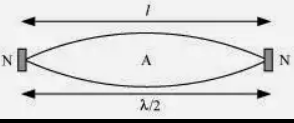The distance between two successive node is λ/2 ∴ l = λ/2 λ = 2l = 2 × l = 2 m The speed of sound in steel is given by the relation:
v = νλ
= 2.53 × 103 × 2
= 5.06 × 103 m/s
= 5.06 km/s

Question 17. A pipe 20 cm long is closed at one end. Which harmonic mode of the pipe is resonantly excited by a 430 Hz source? Will the same source be in resonance with the pipe if both ends are open? (Speed of sound in air is 340 m s–1).

Solution :
First (Fundamental); No
Length of the pipe, l = 20 cm = 0.2 m
Source frequency = nth normal mode of frequency, νn = 430 Hz
Speed of sound, v = 340 m/s
In a closed pipe, the nth normal mode of frequency is given by the relation: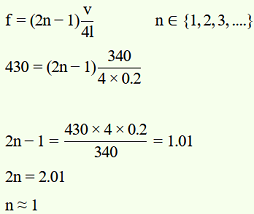Hence, the first mode of vibration frequency is resonantly excited by the given source. In a pipe open at both ends, the nth mode of vibration frequency is given by the relation:
vn = nv/2l
n = 2lvn/v

Question18. Two sitar strings A and B playing the note ‘Ga’ are slightly out of tune and produce beats of frequency 6 Hz. The tension in the string A is slightly reduced and the beat frequency is found to reduce to 3 Hz. If the original frequency of A is 324 Hz, what is the frequency of B?

Solution :
Frequency of string A, fA = 324 Hz
Frequency of string B = fB
Beat’s frequency, n = 6 Hz
Beat's Frequency is given as:

n=|fA​−fB​|
6=|324−fB​|
fB=330Hz or 318Hz

Frequency decreases with a decrease in the tension in a string.

This is because frequency is directly proportional to the square root of tension. It is given as:
v ∝ √T
Hence, the beat frequency cannot be 330 Hz
∴ fB = 318 Hz.

Question19. Explain why (or how):
a. In a sound wave, a displacement node is a pressure antinode and vice versa,
b. Bats can ascertain distances, directions, nature, and sizes of the obstacles without any “eyes”,
c. A violin note and sitar note may have the same frequency, yet we can distinguish between the two notes,
d. Solids can support both longitudinal and transverse waves, but only longitudinal waves can propagate in gases, and
e. The shape of a pulse gets distorted during propagation in a dispersive medium.

Solution :
a. A node (N) is a point where the amplitude of vibration is the minimum and pressure is the maximum.
An antinode (A) is a point where the amplitude of vibration is the maximum and pressure is the minimum.
Therefore, a displacement node is nothing but a pressure antinode, and vice versa.
b. Bats emit ultrasonic waves of large frequencies. When these waves are reflected from the obstacles in their path, they give them the idea about the distance, direction, size and nature of the obstacle.
c. The overtones produced by a sitar and a violin, and the strengths of these overtones, are different. Hence, one can distinguish between the notes produced by a sitar and a violin even if they have the same frequency of vibration.
d. This is because solids have both, the elasticity of volume and elasticity of shape, whereas gases have only the volume elasticity.
e. A sound pulse is a combination of waves of different wavelength. As waves of different λ travel in a dispersive medium with different velocities, therefore, the shape of the pulse gets distorted.

Question20. A train, standing at the outer signal of a railway station blows a whistle of frequency 400 Hz in still air.

1. What is the frequency of the whistle for a platform observer when the train

a. approaches the platform with a speed of 10 m s–1,

b. recedes from the platform with a speed of 10 m s–1?

2.  What is the speed of sound in each case? The speed of sound in still air can be taken as 340 m s–1.

Solution :
1. (a) Frequency of the whistle, ν = 400 Hz
Speed of the train, vT= 10 m/s
Speed of sound, v = 340 m/s
The apparent frequency (v') of the whistle as the train approaches the platform is given by the relation: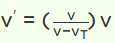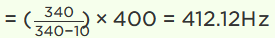(b) The apparent frequency (vn) of the whistle as the train recedes from the platform is given by the relation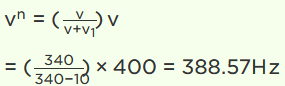(ii) The apparent change in the frequency of sound is caused by the relative motions of the source and the observer. These relative motions produce no effect on the speed of sound. Therefore, the speed of sound in air in both the cases remains the same, i.e., 340 m/s.

Question21. A train, standing in a station-yard, blows a whistle of frequency 400 Hz in still air. The wind starts blowing in the direction from the yard to the station with at a speed of 10 m s–1. What are the frequency, wavelength, and speed of sound for an observer standing on the station’s platform? Is the situation exactly identical to the case when the air is still and the observer runs towards the yard at a speed of 10 m s–1? The speed of sound in still air can be taken as 340 m s–1.

Solution :
For the stationary observer:
Frequency of the sound produced by the whistle, ν = 400 Hz
Speed of sound = 340 m/s
Velocity of the wind, v = 10 m/s
As there is no relative motion between the source and the observer, the frequency of the sound heard by the observer will be the same as that produced by the source, i.e., 400 Hz.
The wind is blowing toward the observer. Hence, the effective speed of the sound increases by 10 units, i.e.,
Effective speed of the sound, ve = 340 + 10 = 350 m/s
The wavelength (λ) of the sound heard by the observer is given by the relation:
λ = ve/v = 350/400 = 0.875 m
For the running observer:
Velocity of the observer, vo = 10 m/s
The observer is moving toward the source. As a result of the relative motions of the source and the observer, there is a change in frequency (v').
This is given by the relation:
Since the air is still, the effective speed of sound = 340 + 0 = 340 m/s
The source is at rest. Hence, the wavelength of the sound will not change, i.e., λ remains 0.875 m.
Hence, the given two situations are not exactly identical.

Question22. A travelling harmonic wave on a string is described by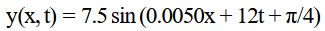a. What are the displacement and velocity of oscillation of a point at x = 1 cm, and t = 1 s? Is this velocity equal to the velocity of wave propagation?
b. Locate the points of the string which have the same transverse displacements and velocity as the x = 1 cm point at t = 2 s, 5 s and 11 s.

Solution :
a. The given harmonic wave is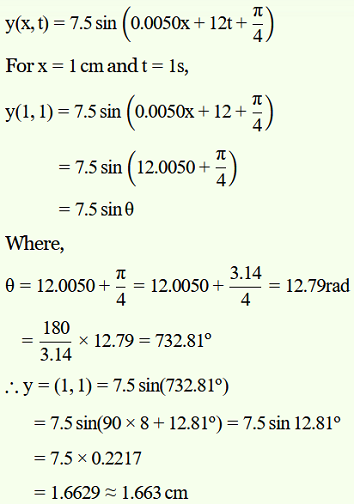The velocity of the oscillation at a given point and times is given as: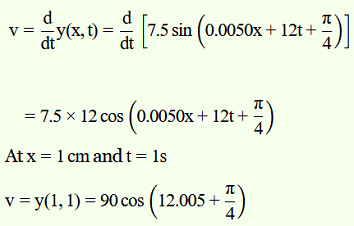= 90 coss (732.81°) = 90 cos (90 × 8 + 12.81°)
= 90 cos (12.81°)
= 90 × 0.975 =87.75 cm/s
Now, the equation of a propagating wave is given by:
y (x, t) = asin (kx + wt + Φ)
where,
k = 2π/λ
∴ λ = 2π/k
and, ω = 2πv
∴ v = ω/2π
Speed, v = vλ = ω/k
where,
k = 0.0050 m-1
∴ v = 12/0.0050 = 2400 cm/s
Hence, the velocity of the wave oscillation at x = 1 cm and t = 1s is not equal to the velocity of the wave propagation.

b. Propagation constant is related to wavelength as:
k = 2π/λ
∴ λ = 2π/k = 2 × 3.14 / 0.0050
= 1256 cm = 12.56 m
Therefore, all the points at distance nλ (n = ±1, ±2, .... and so on), i.e. ± 12.56 m, ± 25.12 m, … and so on for x = 1 cm, will have the same displacement as the x = 1 cm points at t = 2 s, 5 s, and 11 s.

Question 23. A narrow sound pulse (for example, a short pip by a whistle) is sent across a medium.

1. Does the pulse have a definite

a.   frequency,

b.  wavelength,

c.  speed of propagation?

2.  If the pulse rate is 1 after every 20 s, (that is the whistle is blown for a split of second after every 20 s), is the frequency of the note produced by the whistle equal to 1/20 or 0.05 Hz?

Solution :
1. A short pip be a whistle has neither a definite wavelength nor a definite frequency. However, its speed of propagation is fized, being equal to speed of sound in air.
2. No, frequency of the note produced by a whistle is not 1/20 = 0.05 Hz. Rather 0.05 Hz is the frequency of repetition of the short pip of the whistle.

Question 24. One end of a long string of linear mass density 8.0 × 10–3 kg m–1 is connected to an electrically driven tuning fork of frequency 256 Hz. The other end passes over a pulley and is tied to a pan containing a mass of 90 kg. The pulley end absorbs all the incoming energy so that reflected waves at this end have negligible amplitude. At t = 0, the left end (fork end) of the string x = 0 has zero transverse displacement (y = 0) and is moving along positive y-direction. The amplitude of the wave is 5.0 cm. Write down the transverse displacement y as function of x and t that describes the wave on the string.

Solution :
The equation of a travelling wave propagating along the positive y-direction is given by the displacement equation:
y (x, t) = a sin (wt – kx) … (i)
Linear mass density, μ = 8.0 × 10-3 kg m-1 Frequency of the tuning fork, ν = 256 Hz
Amplitude of the wave, a = 5.0 cm = 0.05 m … (ii)
Mass of the pan, m = 90 kg
Tension in the string, T = mg = 90 × 9.8 = 882 N
The velocity of the transverse wave v, is given by the relation: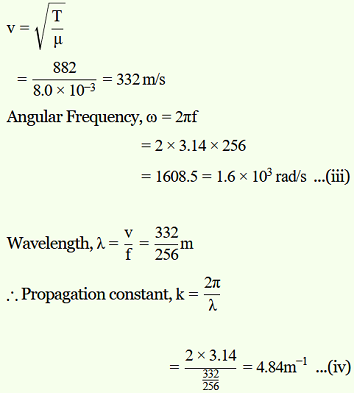Substituting the values from equations (ii), (iii), and (iv) in equation (i), we get the displacement equation:
y (x, t) = 0.05 sin (1.6 × 103t – 4.84 x) m.

Question 25. A SONAR system fixed in a submarine operates at a frequency 40.0 kHz. An enemy submarine moves towards the SONAR with a speed of 360 km h–1. What is the frequency of sound reflected by the submarine? Take the speed of sound in water to be 1450 m s–1.

Solution :
Operating frequency of the SONAR system, ν = 40 kHz
Speed of the enemy submarine, ve = 360 km/h = 100 m/s
Speed of sound in water, v = 1450 m/s
The source is at rest and the observer (enemy submarine) is moving toward it. Hence, the apparent frequency (v') received and reflected by the submarine is given by the relation: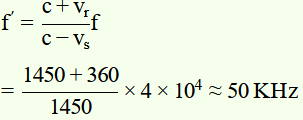Question26. Earthquakes generate sound waves inside the earth. Unlike a gas, the earth can experience both transverse (S) and longitudinal (P) sound waves. Typically the speed of S wave is about 4.0 km s–1, and that of P wave is 8.0 km s–1. A seismograph records P and S waves from an earthquake. The first P wave arrives 4 min before the first S wave. Assuming the waves travel in straight line, at what distance does the earthquake occur?

Solution :
Let vSand vP be the velocities of S and P waves respectively.
Let L be the distance between the epicentre and the seismograph.
We have:
L = vStS          ...(i)
L = vPtP         ...(ii)
Where,
tS and tP are the respective times taken by the S and P waves to reach the seismograph from the epicentre
It is given that:
vP = 8 km/s
vS = 4 km/s
From equations (i) and (ii), we have:
vS tS = vP tP
4tS = 8 tP
tS = 2 tP         ...(iii)
It is also given that:
tS – tP = 4 min = 240 s
2tP – tP = 240
tP = 240
And tS = 2 × 240 = 480 s
From equation (ii), we get:
L = 8 × 240
= 1920 km
Hence, the earthquake occurs at a distance of 1920 km from the seismograph.

Question27. A bat is flitting about in a cave, navigating via ultrasonic beeps. Assume that the sound emission frequency of the bat is 40 kHz. During one fast swoop directly toward a flat wall surface, the bat is moving at 0.03 times the speed of sound in air. What frequency does the bat hear reflected off the wall?

Solution :
Ultrasonic beep frequency emitted by the bat, ν = 40 kHz
Velocity of the bat, vb = 0.03 v
where, v = velocity of sound in air
The apparent frequency of the sound striking the wall is given as: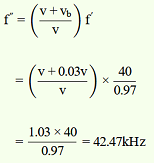This frequency is reflected by the stationary wall (fs​) toward the bat.
The frequency (f′′) of the received sound is given by the relation: